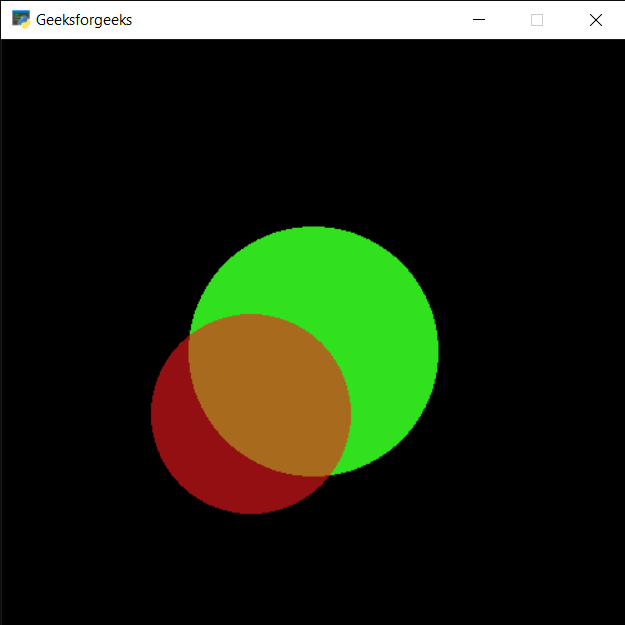Related Articles

# PYGLET – Drawing Circle

• Last Updated : 25 Aug, 2021

In this article we will see how we can draw circles on window in PYGLET module in python. Pyglet is easy to use but powerful library for developing visually rich GUI applications like games, multimedia etc. A window is a “heavyweight” object occupying operating system resources. Windows may appear as floating regions or can be set to fill an entire screen (fullscreen). A circle is a shape consisting of all points in a plane that are a given distance from a given point, the centre; equivalently it is the curve traced out by a point that moves in a plane so that its distance from a given point is constant. Circle is drawn with the help of shapes module in pyglet.
We can create a window with the help of command given below

```# creating a window
window = pyglet.window.Window(width, height, title)```

In order to create window we use Circle method with pyglet.shapes
Syntax : shapes.Circle(x, y, size, color)
Argument : It takes first two integer i.e circle position, third integer as size of circle and forth is tuple i.e color of circle as argument
Return : It returns Circle object

Below is the implementation

## Python3

 `# importing pyglet module``import` `pyglet` `# importing shapes from the pyglet``from` `pyglet ``import` `shapes` `# width of window``width ``=` `500``  ` `# height of window``height ``=` `500``  ` `# caption i.e title of the window``title ``=` `"Geeksforgeeks"``  ` `# creating a window``window ``=` `pyglet.window.Window(width, height, title)` `# creating a batch object``batch ``=` `pyglet.graphics.Batch()`  `# properties of circle``# co-ordinates of circle``circle_x ``=` `250``circle_y ``=` `250` `# size of circle``# color = green``size_circle ``=` `100` `# creating a circle``circle1 ``=` `shapes.Circle(circle_x, circle_y, size_circle, color ``=``(``50``, ``225``, ``30``), batch ``=` `batch)` `# changing opacity of the circle1``# opacity is visibility (0 = invisible, 255 means visible)``circle1.opacity ``=` `250`  `# creating another circle with other properties``# new position = circle1_position - 50``# new size = previous radius -20``# new color = red``circle2 ``=` `shapes.Circle(circle_x``-``50``, circle_y``-``50``, size_circle``-``20``, color ``=``(``250``, ``25``, ``30``), batch ``=` `batch)` `# changing opacity of the circle2``circle2.opacity ``=` `150` `# window draw event``@window``.event``def` `on_draw():``    ` `    ``# clear the window``    ``window.clear()``    ` `    ``# draw the batch``    ``batch.draw()` `# run the pyglet application``pyglet.app.run()`Attention geek! Strengthen your foundations with the Python Programming Foundation Course and learn the basics.

To begin with, your interview preparations Enhance your Data Structures concepts with the Python DS Course. And to begin with your Machine Learning Journey, join the Machine Learning – Basic Level Course

My Personal Notes arrow_drop_up